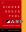Next Functional Programming in Perl 26

# Syntactic Difficulties

• Languages in which HOP is common provide all sorts of handy abbreviations

• For example

```        -- Haskell
half = (/2)
recip = (1/)
divide = (/)
```
• And you probably don't bother to give these names

• In Perl, you get to do

```        my \$half  = sub { \$_ / 2 };
my \$recip = sub { 1 / \$_ };
my \$divide = sub { my \$num = shift; sub { \$num / \$_ } };
```
• Or you get to insert these monstrosities inline

 NextCopyright 2005 M. J. Dominus